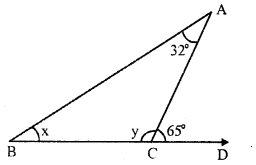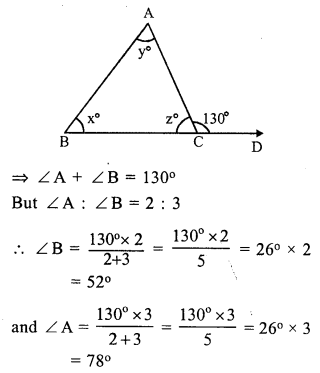# RS Aggarwal Class 7 Solutions Chapter 15 Properties of Triangles Ex 15B

In this chapter, we provide RS Aggarwal Solutions for Class 7 Chapter 15 Properties of Triangles Ex 15B for English medium students, Which will very helpful for every student in their exams. Students can download the latest RS Aggarwal Solutions for Class 7 Chapter 15 Properties of Triangles Ex 15B Maths pdf, free RS Aggarwal Solutions Class 7 Chapter 15 Properties of Triangles Ex 15B Maths book pdf download. Now you will get step by step solution to each question.

### RS Aggarwal Solutions for Class 7 Chapter 15 Properties of Triangles Ex 15B Download PDF

Question 1.
Solution:
In ∆ABC,
∠A = 75°, ∠B = 45°
side BC is produced to Dforming exterior ∠ ACD
Exterior ∠ACD = ∠A + ∠B (Exterior angle is equal to sum of its interior opposite angles)
= 75° + 45° = 120°

Question 2.
Solution:
In ∆ABC, BC is produced to D forming an exterior angle ACD
∠ B = 68°, ∠ A = x°, ∠ ACB = y° and ∠ACD = 130°In triangle,
Exterior angles is equal to sum of its interior opposite angles
∠ACD = ∠A + ∠B
⇒ 130° = x + 68°
⇒ x = 130° – 68° = 62°
But ∠ACB + ∠ACD = 180° (Linear pair)
⇒ y + 130° = 180°
⇒ y = 180° – 130° = 50°
Hence x = 62° and y = 50°

Question 3.
Solution:
In ∆ABC, side BC is produced to D forming exterior angle ACD.
∠ACD = 65°, ∠A = 32°
∠B = x, ∠ACB = yIn a triangle, the exterior angles is equal to the sum of its interior opposite angles
∠ACD = ∠A + ∠B
⇒ 65° = 32° + x
⇒ x = 65° – 32° = 33°
But ∠ ACD + ∠ ACB = 180° (Linear pair)
⇒ 65° + y = 180°
⇒ y = 180°- 65° = 115°
x = 33° and y = 115°

Question 4.
Solution:
In ∆ABC, side BC is produced to D forming exterior ∠ ACD∠ACD = 110°, and ∠A : ∠B = 2 : 3
In a triangle, exterior angles is equal to the sum of its interior opposite angles
⇒ ∠ACD = ∠A + ∠B
⇒ ∠A + ∠B = 110°
But ∠A : ∠B = 2 : 3But ∠A + ∠B + ∠C = 180° (sum of angles of a triangle)
⇒ 44° + 66° + ∠C = 180°
⇒ 110° + ∠C = 180°
⇒ ∠C = 180° – 110° = 70°
Hence ∠ A = 44°, ∠ B = 66° and ∠ C = 70°

Question 5.
Solution:
In ∆ABC, side BC is produced to forming exterior angle ACD.
∠ACD = 100° and ∠A = ∠B
Exterior angle of a triangle is equal to the sum of its interior opposite angles.∠ACD = ∠A + ∠B But ∠A = ∠B
∠A + ∠A = ∠ACD = 100°
⇒ 2 ∠A = 100°
⇒ ∠A = 50°
∠B = ∠A = 50°
But ∠A + ∠B + ∠ ACB = 180° (sum of angles of a triangle)
⇒ 50° + 50° + ∠ ACB = 180°
⇒ 100° + ∠ ACB = 180°
⇒ ∠ ACB = 180° – 100° = 80°
Hence ∠ A = 50°, ∠ B = 50° and ∠ C = 80°

Question 6.
Solution:
In ∆ABC, side BC is produced to D From D, draw a line meeting AC at E so that ∠D = 40°
∠A = 25°, ∠B = 45°
In ∆ABC,Exterior ∠ACD = ∠A + ∠B = 25° + 45° = 70°
Again, in ∆CDE,
Exterior ∠ AED = ∠ ECD + ∠ D = ∠ACD + ∠D = 70° + 40° = 110°
Hence ∠ACD = 70° and ∠AED = 110°

Question 7.
Solution:
In ∆ABC, sides BC is produced to D and BA to E
∠CAD = 50°, ∠B = 40° and ∠ACB = 100°
∠ ACB + ∠ ACD = 180° (Linear pair)
⇒ 100° + ∠ ACD = 180°
⇒ ∠ ACD = 180° – 100° = 80°In ∆ACD,
∠ CAD + ∠ ACD + ∠ ADC = 180° (sum of angles of a triangle)
⇒ 50° + 80° + ∠ ADC = 180°
⇒ 130° + ∠ ADC = 180°
⇒ ∠ ADC = 180° – 130° = 50°
Now, in ∆ABD, BA is produced to E
Exterior ∠DAE = ∠ACD + ∠ADC = 80° + 50° = 130°
Hence ∠ ACD = 80°, ∠ ADC = 50° and ∠DAE = 130°

Question 8.
Solution:
In ∆ABC, BC is produced to D forming exterior ∠ ACD∠ACD = 130°, ∠A = y°, ∠B = x° and ∠ACB = z°.
x : y = 2 : 3
Now, in ∆ABC,
Exterior ∠ACD = ∠A + ∠BBut ∠A + ∠B + ∠ACB = 180° (sum of angles of a triangle)
⇒ 78° + 52° + ∠ACB = 180°
⇒ 130° + ∠ACB = 180°
⇒ ∠ACB = 180° – 130°
⇒ ∠ACB = 50°
⇒ ∠ = 50°
Hence x = 52°, y = 78° and z = 50°

All Chapter RS Aggarwal Solutions For Class 7 Maths

—————————————————————————–

All Subject NCERT Exemplar Problems Solutions For Class 7

All Subject NCERT Solutions For Class 7

*************************************************

I think you got complete solutions for this chapter. If You have any queries regarding this chapter, please comment on the below section our subject teacher will answer you. We tried our best to give complete solutions so you got good marks in your exam.

If these solutions have helped you, you can also share rsaggarwalsolutions.in to your friends.